Courses

# NCERT Solutions(Part - 2) - Symmetry Class 7 Notes | EduRev

## Class 7 : NCERT Solutions(Part - 2) - Symmetry Class 7 Notes | EduRev

The document NCERT Solutions(Part - 2) - Symmetry Class 7 Notes | EduRev is a part of the Class 7 Course Mathematics (Maths) Class 7.
All you need of Class 7 at this link: Class 7

Exercise 14.3

Question 1:

Name any two figures that have both line symmetry and rotational symmetry.

Circle and Square.

Question 2:

Draw, wherever possible, a rough sketch of:

(i) a triangle with both line and rotational symmetries of order more than 1.

(ii) a triangle with only line symmetry and no rotational symmetry of order more than 1.

(iii) a quadrilateral with a rotational symmetry of order more than 1 but not a line symmetry.

(iv) a quadrilateral with line symmetry but not a rotational symmetry of order more than 1.

(i) An equilateral triangle has both line and rotational symmetries of order more than 1.

Line symmetry: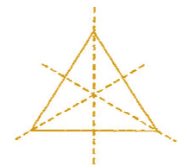Rotational symmetry: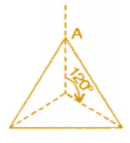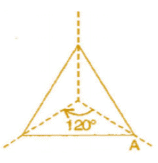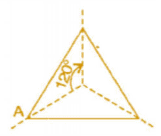(ii) An isosceles triangle has only one line of symmetry and no rotational symmetry of order more than 1.

Line symmetry: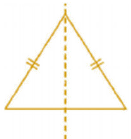Rotational symmetry: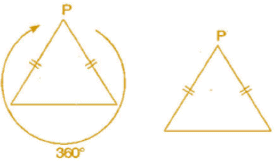(iii) It is not possible because order of rotational symmetry is more than 1 of a figure, most acertain the line of symmetry.

(iv) A trapezium which has equal non-parallel sides, a quadrilateral with line symmetry but not a rotational symmetry of order more than 1.

Line symmetry: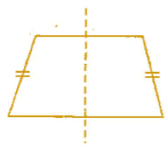Rotational symmetry: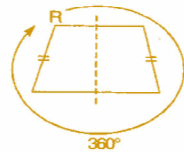Question 3:

In a figure has two or more lines of symmetry, should it have rotational symmetry of order more than 1?

Yes, because every line through the centre forms a line of symmetry and it has rotational symmetry around the centre for every angle.

Question 4:

Fill in the blanks:

 Shape Centre of Rotation Order of Rotation Angle of Rotation Square Rectangle Rhombus Equilateral triangle Regular hexagon Circle Semi-circle

 Shape Centre of Rotation Order of Rotation Angle of Rotation Square Intersecting point of diagonals. 4 90° Rectangle Intersecting point of diagonals. 2 180° Rhombus Intersecting point of diagonals. 2 180° Equilateraltriangle Intersecting point of medians. 3 120° Regularhexagon Intersecting point of diagonals. 6 60° Circle Centre infinite At every point Semi-circle Mid-point of diameter 1 360°

Question 5:

Name the quadrilateral which has both line and rotational symmetry of order more than 1.

Square has both line and rotational symmetry of order more than 1.

Line symmetry: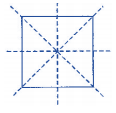Rotational symmetry: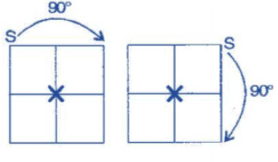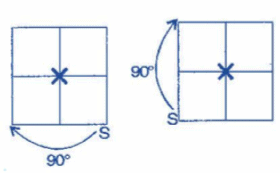Question 6:

After rotating by 60o about a centre, a figure looks exactly the same as its original position. At what other angles will this happen for the figure?

Other angles will be 120°, 180°,240°,300°,360°.

For 60° rotation:

It will rotate six times.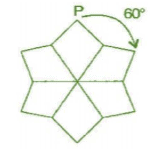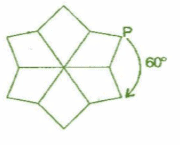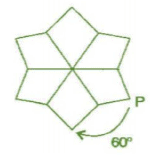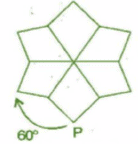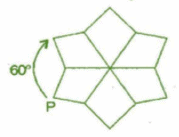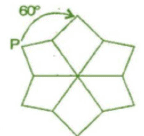For 120° rotation:

It will rotate three times.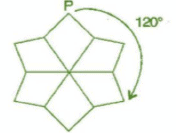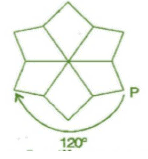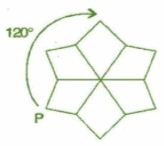For 180° rotation:

It will rotate two times.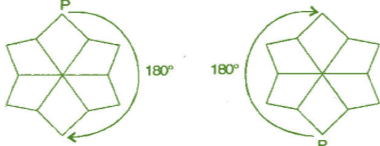For 360° rotation:

It will rotate one time.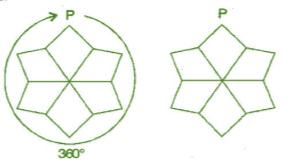Question 7:

Can we have a rotational symmetry of order more than 1 whose angle of rotation is:

(i) 45o
(ii) 17o

(i) If tiie angle of rotation is 45°, then symmetry of order is possible and would be 8 rotations.
(ii) If the angle of rotational is 17°, then symmetry o f order is not possible because 360° is not complete divided by 17°.

Offer running on EduRev: Apply code STAYHOME200 to get INR 200 off on our premium plan EduRev Infinity!

## Mathematics (Maths) Class 7

161 videos|237 docs|45 tests

,

,

,

,

,

,

,

,

,

,

,

,

,

,

,

,

,

,

,

,

,

;